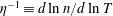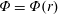Hostname: page-component-797576ffbb-k7d4m Total loading time: 0 Render date: 2023-12-10T19:58:25.069Z Has data issue: false Feature Flags: { "corePageComponentGetUserInfoFromSharedSession": true, "coreDisableEcommerce": false, "useRatesEcommerce": true } hasContentIssue false

## Abstract

Impurity temperature screening is a favourable neoclassical phenomenon involving an outward radial flux of impurity ions from the core of fusion devices. Quasisymmetric magnetic fields lead to intrinsically ambipolar neoclassical fluxes that give rise to temperature screening for low enough$\unicode[STIX]{x1D702}^{-1}\equiv d\ln n/d\ln T$. In contrast, neoclassical fluxes in generic stellarators will depend on the radial electric field, which is predicted to be inward for ion-root plasmas, potentially leading to impurity accumulation. Here, we examine the impurity particle flux in a number of approximately quasisymmetric stellarator configurations and parameter regimes while varying the amount of symmetry breaking in the magnetic field. For the majority of this work, neoclassical fluxes have been obtained using the SFINCS drift-kinetic equation solver with electrostatic potential$\unicode[STIX]{x1D6F7}=\unicode[STIX]{x1D6F7}(r)$, where$r$ is a flux-surface label. Results indicate that achieving temperature screening is possible, but unlikely, at low-collisionality reactor-relevant conditions in the core. Thus, the small departures from symmetry in nominally quasisymmetric stellarators are large enough to significantly alter the neoclassical impurity transport. A further look at the magnitude of these fluxes when compared to a gyro-Bohm turbulence estimate suggests that neoclassical fluxes are small in configurations optimized for quasisymmetry when compared to turbulent fluxes.

Type
Research Article

## Access options

Get access to the full version of this content by using one of the access options below. (Log in options will check for institutional or personal access. Content may require purchase if you do not have access.)

## References

Abel, I. G., Plunk, G. G., Wang, E., Barnes, M., Cowley, S. C., Dorland, W. & Schekochihin, A. A. 2013 Multiscale gyrokinetics for rotating tokamak plasmas: fluctuations, transport and energy flows. Rep. Prog. Phys. 76.CrossRefGoogle ScholarPubMed
Allmaier, K., Kasilov, S. V., Kernbichler, W. & Leitold, G. O. 2008 Variance reduction in computations of neoclassical transport in stellarators using a$\unicode[STIX]{x1D6FF}f$ method. Phys. Plasmas 15.CrossRefGoogle Scholar
Anderson, F. S. B. et al. 1995 The Helically Symmetric Experiment, (HSX) goals, design and status. Fusion Tech. 27, 273277.CrossRefGoogle Scholar
Bader, A., Drevlak, M., Anderson, D. T., Faber, B. J., Hegna, C. C., Likin, K. M., Schmitt, J. C. & Talmadge, J. N. 2019 Stellarator equilibria with reactor relevant energetic particle losses. J. Plasma Phys. 85.CrossRefGoogle Scholar
Beidler, C. D. et al. 2008 Transport modeling for W7-X on the basis of W7-AS experimental results. In Proceedings of the 17th International Toki Conference and 16th International Stellarator/Heliotron Workshop, Toki.Google Scholar
Beidler, C. D. et al. 2011 Benchmarking of the mono-energetic transport coefficients-results from the International Collaboration on Neoclassical Transport in Stellarators (ICNTS). Nucl. Fusion 51.CrossRefGoogle Scholar
Boozer, A. H. 1981 Plasma equilibrium with rational magnetic surfaces. Phys. Fluids 24, 19992003.CrossRefGoogle Scholar
Boozer, A. H. 1982 Establishment of magnetic coordinates for a given magnetic field. Phys. Fluids 25, 520521.CrossRefGoogle Scholar
Boozer, A. H. 1983 Transport and isomorphic equilibria. Phys. Fluids 26, 496499.CrossRefGoogle Scholar
Buller, S., Smith, H. M., Helander, P., Mollén, A., Newton, S. L. & Pusztai, I. 2018 Collisional transport of impurities with flux-surface varying density in stellarators. J. Plasma Phys. 84.CrossRefGoogle Scholar
Calvo, I., Parra, F. I., Velasco, J. L., Alonso, J. A. & García-Regaña, J. M. 2018 Stellarator impurity flux driven by electric fields tangent to magnetic surfaces. Nucl. Fusion 58 (12), 124005.CrossRefGoogle Scholar
Canik, J. M., Anderson, D. T., Anderson, F. S. B., Clark, C., Likin, K. M., Talmadge, J. N. & Zhai, K. 2007 Reduced particle and heat transport with quasisymmetry in the Helically Symmetric Experiment. Phys. Plasmas 14.CrossRefGoogle Scholar
Cary, J. R. & Shasharina, S. G. 1997 Helical plasma confinement devices with good confinement properties. Phys. Rev. Lett. 78, 674677.CrossRefGoogle Scholar
Connor, J. W. 1973 The neo-classical transport theory of a plasma with multiple ion species. Plasma Phys. 15, 765782.CrossRefGoogle Scholar
Connor, J. W. 1988 Invariance principles and plasma confinement. Plasma Phys. Control. Fusion 30 (619).CrossRefGoogle Scholar
Engelmann, F. 1990 Extrapolation of tokamak energy confinement to next step devices. Plasma Phys. Control. Fusion 32 (1101).CrossRefGoogle Scholar
Galeev, A. A. & Sagdeev, R. Z. 1968 Transport phenomena in a collisionless plasma in a toroidal magnetic system. Sov. Phys. JETP 26, 233240.Google Scholar
Garabedian, P. R. 1996 Stellarators with the magnetic symmetry of a tokamak. Phys. Plasmas 3, 24832485.CrossRefGoogle Scholar
García-Regaña, J. M. et al. 2017 Electrostatic potential variation on the flux surface and its impact on impurity transport. Nucl. Fusion 57.CrossRefGoogle Scholar
Garren, D. A. & Boozer, A. H. 1991 Existence of quasihelically symmetric stellarators. Phys. Fluids B 3, 28222834.CrossRefGoogle Scholar
Geiger, B. et al. 2019 Observation of anomalous impurity transport during low-density experiment in W7-X with laser blow-off injections of iron. Nucl. Fusion 59 (046009).CrossRefGoogle Scholar
Hazeltine, R. D. 1973 Recursive derivation of drift-kinetic equation. Plasma Phys. 15, 7780.CrossRefGoogle Scholar
Helander, P. et al. 2012 Stellarator and tokamak plasmas: a comparison. Plasma Phys. Control. Fusion 54.CrossRefGoogle Scholar
Helander, P., Newton, S. L., Mollén, A. & Smith, H. M. 2017 Impurity transport in a mixed-collisionality stellarator plasma. Phys. Rev. Lett. 118.CrossRefGoogle Scholar
Helander, P. 2007 On rapid plasma rotation. Phys. Plasmas 14.CrossRefGoogle Scholar
Helander, P. 2014 Theory of plasma confinement in non-axisymmetric magnetic fields. Rep. Prog. Phys. 77.CrossRefGoogle ScholarPubMed
Helander, P. & Sigmar, D. J. 2002 Collisional Transport in Magnetized Plasmas. Cambridge University Press.Google Scholar
Hender, T. 1988 Studies of a flexible heliac configuration. Fusion Tech. 13, 521535.CrossRefGoogle Scholar
Henneberg, S. A., Drevlak, M., Nührenberg, C., Beidler, C. D., Turkin, Y., Loizu, J. & Helander, P. 2019 Properties of a new quasi-axisymmetric configuration. Nucl. Fusion 59.CrossRefGoogle Scholar
Hirsch, M. 2008 Major results from the stellarator Wendelstein 7-AS. Plasma Phys. Control. Fusion 50 (5).CrossRefGoogle Scholar
Hirshman, S. P., Shaing, K. C., van Rij, W. I., Beasley, C. O. & Crume, E. C. 1986a Plasma transport coefficients for nonsymmetric toroidal confinement systems. Phys. Fluids 29.CrossRefGoogle Scholar
Hirshman, S. P. 1978 The ambipolarity paradox in toroidal diffusion, revisited. Nucl. Fusion 18.CrossRefGoogle Scholar
Hirshman, S. P., van Rij, W. I. & Merkel, P. 1986b Three-dimensional free boundary calculations using a spectral Green’s function method. Comput. Phys. Comm. 43, 143155.CrossRefGoogle Scholar
Ida, K. et al. 2009 Observation of an impurity hole in a plasma with an ion internal transport barrier in the Large Helical Device. Phys. Plasmas 16.CrossRefGoogle Scholar
Igitkhanov, Y., Polunovsky, E. & Beidler, C. D. 2006 Impurity dynamics in nonaxisymmetric plasmas. Fusion Sci. Tech. 50, 268275.CrossRefGoogle Scholar
Kernbichler, W., Kasilov, S. V., Leitold, G. O., Nemov, V. V. & Allmaier, L. 2008 Recent progress in NEO-2 – a code for neoclassical transport computations based on field line tracing. Plasma Fusion Res. 3.CrossRefGoogle Scholar
Klinger, T. et al. 2017 Performance and properties of the first plasmas of Wendelstein 7-X. Plasma Phys. Control. Fusion 59.CrossRefGoogle Scholar
Landreman, M., Smith, H. M., Mollén, A. & Helander, P. 2014 Comparison of particle trajectories and collision operators for collisional transport in nonaxisymmetric plasmas. Phys. Plasmas 21.CrossRefGoogle Scholar
Liu, H. et al. 2018 Magnetic configuration and modular coil design for the Chinese First Quasi-Axisymmetric Stellarator. Plasma Fusion Res. 13.CrossRefGoogle Scholar
Maaßberg, H., Beidler, C. D. & Simmet, E. E. 1999 Density control problems in large stellarators with neoclassical transport. Plasma Phys. Control. Fusion 41, 11351153.CrossRefGoogle Scholar
Mollén, A., Landreman, M., Smith, H. M., García-Regaña, J. M. & Nunami, M. 2018 Flux-surface variations of the electrostatic potential in stellarators: impact on the radial electric field and neoclassical impurity transport. Plasma Phys. Control. Fusion 60 (084001).CrossRefGoogle Scholar
Mynick, H. 1984 Calculation of the poloidal ambipolar field in a stellarator and its effect on transport. Phys. Fluids 27, 20862092.CrossRefGoogle Scholar
Mynick, H. E., Boozer, A. H. & Ku, L. P. 2006 Improving confinement in quasi-axisymmetric stellarators. Phys. Plasmas 13, 15.CrossRefGoogle Scholar
Najmabadi, F. 2008 The ARIES-CS compact stellarator. Fusion Sci. Tech. 54.CrossRefGoogle Scholar
Nemov, V. V., Kasilov, S. V., Kernbichler, W. & Heyn, M. F. 1999 Evaluation of$1/\unicode[STIX]{x1D708}$ neoclassical transport in stellarators. Phys. Plasmas 6, 4622–2632.CrossRefGoogle Scholar
Nührenberg, J. & Zille, R. 1988 Quasi-helically symmetric toroidal stellarators. Phys. Lett. A 129, 113117.CrossRefGoogle Scholar
Pablant, N. A. et al. 2018 Core radial electric field and transport in Wendelstein 7-X plasmas. Phys. Plasmas 25.CrossRefGoogle Scholar
Post, R. F. 1961 Impurity radiation losses from a high temperature plasma. J. Nucl. Energy 3 (4), 273286.CrossRefGoogle Scholar
Pytte, A. & Boozer, A. H. 1981 Neoclassical transport in helically symmetric plasmas. Phys. Fluids 24, 8892.CrossRefGoogle Scholar
van Rij, W. I. & Hirshman, S. P. 1989 Variational bounds for transport coefficients in three-dimensional toroidal plasmas. Phys. Fluids B 1, 563569.CrossRefGoogle Scholar
Rosenbluth, M. N., Hazeltine, R. D. & Hinton, F. L. 1972 Plasma transport in toroidal confinement systems. Phys. Fluids 15, 116140.CrossRefGoogle Scholar
Rutherford, P. H. 1970 Collisional diffusion in an axisymmetric torus. Phys. Fluids 14, 482489.CrossRefGoogle Scholar
Rutherford, P. H. 1974 Impurity transport in the Pfirsch-Schlüter regime. Phys. Fluids 17, 17821784.CrossRefGoogle Scholar
Shaing, K. C. 1986 Plasma flows and radial electric field in nonaxisymmetric toroidal plasmas. Phys. Fluids 29, 22312234.CrossRefGoogle Scholar
Shimizu, A. et al. 2018 Configuration property of the Chinese first quasi-axisymmetric stellarator. Plasma Fusion Res. 13.CrossRefGoogle Scholar
Sugama, H., Watanabe, T.-H., Nunami, M. & Nishimura, S. 2011a Quasisymmetric toroidal plasmas with large mean flows. Phys. Plasmas 18.CrossRefGoogle Scholar
Sugama, H., Watanabe, T. H., Nunami, M. & Nishimura, S. 2011b Momentum balance and radial electric fields in axisymmetric and nonaxisymmetric toroidal plasmas. Plasma Phys. Control. Fusion 53 (024004).CrossRefGoogle Scholar
Tribaldos, V. 2001 Monte Carlo estimation of neoclassical transport for the TJ-II stellarator. Phys. Plasmas 8, 12291239.CrossRefGoogle Scholar
Turkin, Y., Beidler, C. D., Maaßberg, H., Murakami, S., Tribaldos, V. & Wakasa, A. 2011 Neoclassical transport simulations for stellarators. Phys. Plasmas 18.CrossRefGoogle Scholar
Velasco, J. L. et al. 2017 Moderation of neoclassical impurity accumulation in high temperature plasmas of helical devices. Nucl. Fusion 57.CrossRefGoogle Scholar
Wade, M. R., Houlberg, W. A. & Baylor, L. R. 2000 Experimental confirmation of impurity convection driven by the ion-temperature gradient in toroidal plasmas. Phys. Rev. Lett. 84, 282285.CrossRefGoogle ScholarPubMed
Waltz, R. E., DeBoo, J. C. & Rosenbluth, M. N. 1990 Magnetic-field scaling of dimensionally similar tokamak discharges. Phys. Rev. Lett. 65 (19).CrossRefGoogle ScholarPubMed
Zarnstorff, M. C. et al. 2001 Physics of the compact advanced stellarator NCSX. Plasma Phys. Control. Fusion 43, A237A249.CrossRefGoogle Scholar